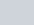Two arithmetic progression have the same common difference.
Question:

Two arithmetic progression have the same common difference. The difference between their 100th terms is 100, What is the difference between their 1000th terms?

Solution:

Here, we are given two A.P sequences which have the same common difference. Let us take the first term of one A.P. as a and of other A.P. as a’

Also, it is given that the difference between their 100th terms is 100.

We need to find the difference between their 100th terms

So, let us first find the 100th terms for both of them.

Now, as we know,

$a_{n}=a+(n-1) d$

So, for 100th term of first A.P. (n = 100),

$a_{100}=a+(100-1) d$

$=a+99 d$

Now, for 100th term of second A.P. (n = 100),

$a_{100}^{\prime}=a^{\prime}+(100-1) d$

$=a^{\prime}+99 d$

Now, we are given,

$a_{100}-a_{100}^{\prime}=100$

On substituting the values, we get,

$a+99 d-a^{\prime}-99 d=100$

$a-a^{\prime}=100$ …….(1)

Now, we need the difference between the 1000th terms of both the A.P.s

So, for 1000th term of first A.P. (n = 1000),

$a_{1000}=a+(1000-1) d$

$=a+999 d$

Now, for 1000th term of second A.P. (n = 1000),

$a_{1600}^{\prime}=a^{\prime}+(1000-1) d$

$=a^{\prime}+999 d$

So,

$a_{1000}-a_{1000}^{\prime}=(a+999 d)-\left(a^{\prime}+999 d\right)$

$=a+999 d-a^{\prime}-999 d$

$=a-a^{\prime}$

$=100$    (Using 1 )

Therefore, the difference between the 1000th terms of both the arithmetic progressions will be.